# Perimeter

Print Rate 0 stars Common Core
Lesson size:
Message preview:
Someone you know has shared lesson with you:

To play this lesson, click on the link below:

https://www.turtlediary.com/lesson/perimeter.html

Hope you have a good experience with this site and recommend to your friends too.

Login to rate activities and track progress.
Login to rate activities and track progress.

The perimeter is the distance around an object. It is calculated by adding together all the lengths of an object.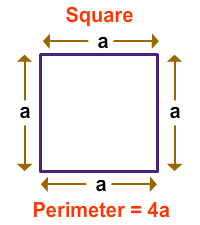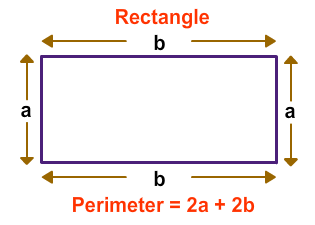What is a Polygon?

- It is a closed plane shape or figure

- It has straight sides

Some of the polygons are as follows: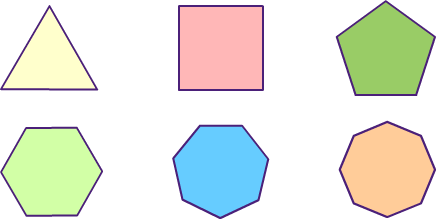Example 1

• Find the perimeter of the given square.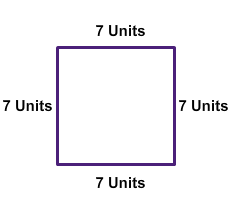To find the perimeter of the polygon, you would just add the length of all sides together.

So, the perimeter of this square is 7 + 7 + 7 + 7= 28 units

Remember: Perimeter of a polygon = sum of the length of all sides

Example 2

• Ants are walking around your picnic mat as shown in the image given below. Measure the sides of the picnic mat and add them to find out how far the ants walked.The length and the width of the mat is 8 feet and 6 feet respectively. To find the perimeter, we need to add all the sides.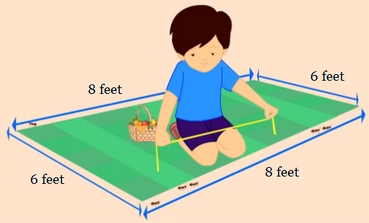The perimeter will be:

8 + 6 + 8 + 6 = 28

So, the perimeter of the picnic mat is 28 feet.

Example 3

• While making a card for your parents you want to put yarn around the card. Measure the sides to find out how much yarn is required.The length and the width of the card is 6 inches and 4 inches respectively.The Perimeter of the card is:

6 + 4 + 4 + 6 = 20

So, 20 inches of yarn is required for the card.

## Perimeter

• The perimeter is the distance around an object. It is calculated by adding together all the lengths of an object.
• The perimeter of a square is 4a.
•• The perimeter of a rectangle is 2a + 2b
•## Similar Lessons

Become premium member to get unlimited access.